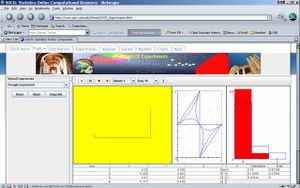# SOCR EduMaterials Activities Triangles

## SOCR Educational Materials - Activities - SOCR Triangle Experiment Assignment

Activity Goal: To understand how simple it is sometimes to find complicated probabilities by simulation. We will use the following question: what is the probability that if we select two points at random from the interval [0,1], the resulting three pieces may form an obtuse triangle?

Review of geometry: To review some geometry, an acute triangle is a triangle in which all three angles are acute angles (< \(90^o\)). A triangle is abtuse if one of its angles is abtuse (> \(90^o\)). An obtuse triangle is a triangle in which one of the angles is an obtuse angle. A triangle can be acute, obtuse or right.

To understand the questions below, consider that the interval [0,1] is the interval given below and X and Y are as indicated. I picked up the resulting three lines with length [0,X], [X,Y] and [Y,1] and tried to shape them into a triangle, without success. But sometimes I may be able to get either an acute triangle or an obtuse triangle. How often do I get each or none?

• Experiment Setting: Go to the Triangles Experiment applet at the following website:

http://socr.stat.ucla.edu/htmls/SOCR_Experiments.html. Select the Triangle Experiment from the drop-down list on the top-left.In this activity, two points are chosen at random in the interval [0,1]; X denotes the first point chosen and Y denotes the second point chosen. U gives the type of triangle that can be formed from the three sub-intervals: U=0, the pieces do not form a triangle; U=1, the pieces form an obtuse triangle; U=2, the pieces form an acute triangle. The blue distribution on the top of the right hand side gives you the theoretical probability. So does the table right below it. On the left hand side on the yellow background, you will see the sublines determined by the X and Y chosen, and whether they form a triangle. The graph right next to it shows the X and the Y.

• Exercise 1: According to the theoretical probabilities, what is the probability of getting an obtuse triangle? * What is the theoretical probability of getting an acute triangle?
• Exercise 2: Choose X and Y at random once. To do that, click on the run one-step button ( ). Which X did you get ? __________________Which Y did you get? __________Is that the same as the value of V? What is the value of V ? ____________. Do they form any kind of triangle?

• Exercise 3: If they form a triangle, which kind? What you just did is one repetition of the experiment. You will see on the blue chart with the theoretical distribution that there is also a red distribution. What does the red look like? What does the table for “data” right below say? How does the data table (and graph) compare with the empirical? Print the page you got (with table, triangle, etc..) Attach to this handout. Of course, one repetition of the experiment will not give us the true probability. To approximate the true probability, we will need to repeat the experiment many times. So let’s do that.
• Exercise 4: Repeat the above until you get 10 repetitions. How many of those 10 repetitions gave you abtuse triangles. What proportion of the 10 repetitions gave you obtuse triangles? What happened to the empirical distribution? Does it look more like the theoretical one? Print out of the page after you got the 10 replications.
• Excersize 5: Ten repetitions is still not enough. So we can try to do it many more times. We need to repeat the same experiment many times to see how often we get acute triangles, obtuse triangles, or no triangles at all. Make Stop 10,000 to repeat the experiments 10,000 times, and update every 1,000 times. Print out what you got and comment on the similarities of the theoretical and the empirical distributions.